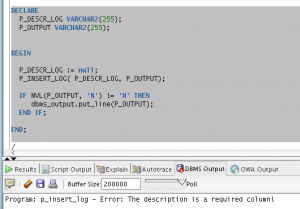# PL/SQL For Practitioners – #5 Procedures

Hi folks,

A procedure is a subprogram that performs a specific action. This feature is extremely important and vastly that we can use when developing in PL/SQL. Before invoking a procedure, you must declare and define it. You can either declare it first (with procedure_declaration) and then define it later in the same block, subprogram, or package (with procedure_definition) or declare and define it at the same time (with procedure_definition).

## Syntax

```create or replace procedure <Name> (<parameter list>)
as
--local variable declaration
begin
--code section
exceptions --optional
--handlers
end;```

## Example 1

The following procedure is a quite simple example and it is responsible for inserting new registers to the log table. The file create_table_it_tutorial_log create_table_it_tutorial_log

### Procedure

```CREATE OR REPLACE
PROCEDURE p_insert_log (p_descr_log varchar2) AS

v_new_id number;

BEGIN

select nvl(max(id), 0) + 1 into v_new_id from it_tutorial_log;

insert into it_tutorial_log (id, descr_log, date_log, user_log) values (v_new_id, p_descr_log, sysdate, user);

END p_insert_log;
```

### Execution

```DECLARE
P_DESCR_LOG VARCHAR2(200);
BEGIN

P_DESCR_LOG := 'FIRST PROCEDURE TEST';

P_INSERT_LOG( P_DESCR_LOG );

END;
```

## Example 2 – Adding exception handling

For more details about exception handling please check out my post below

PL/SQL For Practitioners – #4 Exception Handling

### Procedure

```create or replace
PROCEDURE p_insert_log (p_descr_log varchar2,
p_msg_return OUT varchar2) AS

v_new_id NUMBER;
v_local_except EXCEPTION;
v_error_msg VARCHAR2(255);

BEGIN

select nvl(max(id), 0) + 1 into v_new_id from it_tutorial_log;

IF NVL(p_descr_log, 'N') = 'N' THEN
v_error_msg := 'The description is a required column!';
raise v_local_except;
END IF;

insert into it_tutorial_log (id, descr_log, date_log, user_log) values (v_new_id, p_descr_log, sysdate, user);

EXCEPTION
WHEN v_local_except THEN
p_msg_return := 'Program: p_insert_log - Error: ' || v_error_msg;

END p_insert_log;
```

### Execution

```DECLARE
P_DESCR_LOG VARCHAR2(255);
P_OUTPUT VARCHAR2(255);
BEGIN
P_DESCR_LOG := null;
P_INSERT_LOG( P_DESCR_LOG, P_OUTPUT);

IF NVL(P_OUTPUT, 'N') != 'N' THEN
dbms_output.put_line(P_OUTPUT);
END IF;
END;```This post is part of a series about the most common structures in PL/SQL. You can check the rest of them in the link below.

PL/SQL for real world – 8 features that you have to know